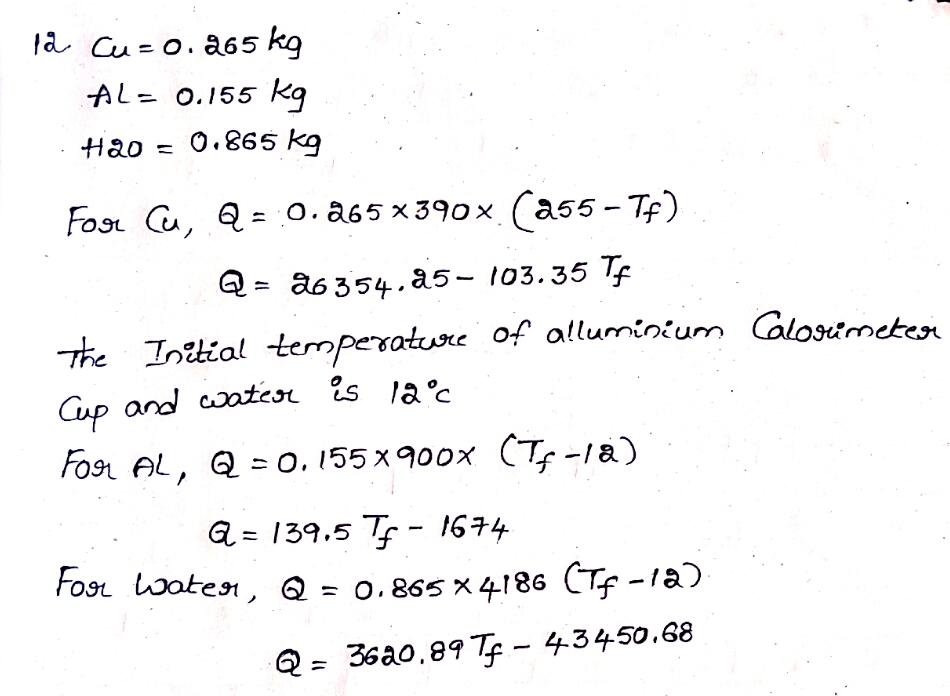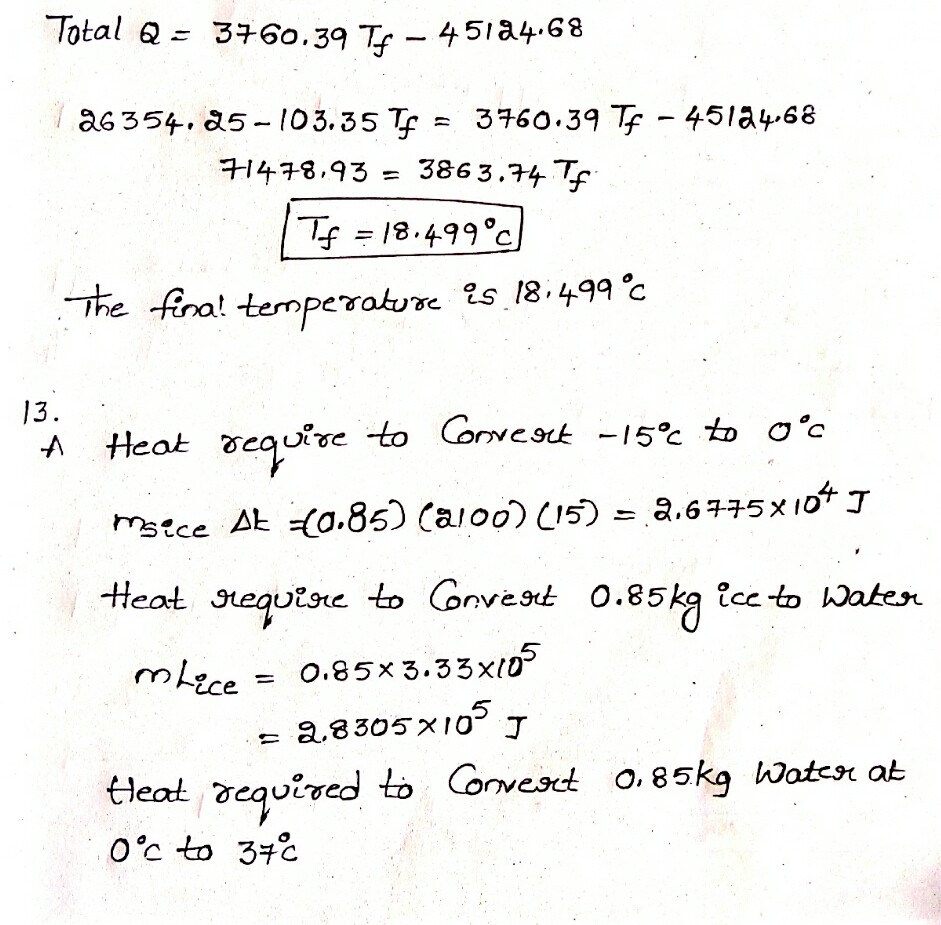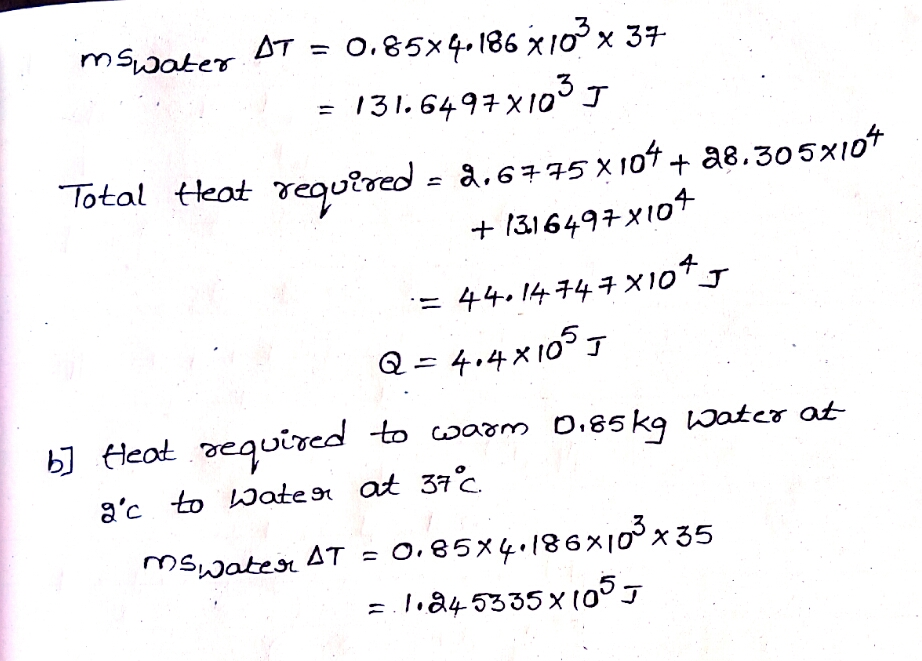In: Physics

# Chapter #14 Question #12, The value of specific heat for copper is 390 J/kg⋅C∘, for aluminun...

Chapter #14

Question #12,

The value of specific heat for copper is 390 J/kg⋅C∘, for aluminun is 900 J/kg⋅C∘, and for water is 4186 J/kg⋅C∘.
Part A
What will be the equilibrium temperature when a 265 g block of copper at 255 ∘C is placed in a 155 g aluminum calorimeter cup containing 865 g of water at 12.0 ∘C?

T =

Question #13

High-altitude mountain climbers do not eat snow, but always melt it first with a stove. To see why, calculate the energy absorbed from a climber's body under the following conditions. The specific heat of ice is 2100 J/kg⋅C∘, the latent heat of fusion is 333 kJ/kg, the specific heat of water is 4186 J/kg⋅C∘.
Part A
Calculate the energy absorbed from a climber's body if he eats 0.85 kg of -15∘C snow which his body warms to body temperature of 37∘C.
Express your answer to two significant figures and include the appropriate units.

Q1 =
Part B
Calculate the energy absorbed from a climber's body if he melts 0.85 kg of -15∘C snow using a stove and drink the resulting 0.85 kg of water at 2∘C, which his body has to warm to 37∘C.
Express your answer to two significant figures and include the appropriate units.

Q2 =

## Solutions

##### Expert Solution## Related Solutions

##### The value of specific heat for copper is 390 J/kg⋅C∘, for aluminun is 900 J/kg⋅C∘, and...
The value of specific heat for copper is 390 J/kg⋅C∘, for aluminun is 900 J/kg⋅C∘, and for water is 4186 J/kg⋅C∘. What will be the equilibrium temperature when a 225 g block of copper at 245 ∘C is placed in a 155 g aluminum calorimeter cup containing 835 g of water at 14.0 ∘C ? Express your answer using three significant figures.
##### The value of specific heat for copper is 390 J/kg⋅C∘, for aluminun is 900 J/kg⋅C∘, and...
The value of specific heat for copper is 390 J/kg⋅C∘, for aluminun is 900 J/kg⋅C∘, and for water is 4186 J/kg⋅C∘. Part A What will be the equilibrium temperature when a 275 g block of copper at 245 ∘C is placed in a 135 g aluminum calorimeter cup containing 855 g of water at 15.0 ∘C? Express your answer using three significant figures.' T=  ∘C
##### 1. A 5.88 kg piece of granite with a specific heat of 0.803 J g-1 °C-1...
1. A 5.88 kg piece of granite with a specific heat of 0.803 J g-1 °C-1 and a temperature of 85.1 °C is placed into 2.00 L of water at 19.0 °C. When the granite and water come to the same temperature, what will the temperature be? 2. The combustion of methane (the chief component of natural gas) follows the equation: CH4(g) + 2O2(g) → CO2(g) + 2H2O(g) ∆H° for this reaction is -802.3 kJ. How many grams of methane...
##### I just need to find the specific heat of copper, steel and aluminum. Experiment 12 Specific...
I just need to find the specific heat of copper, steel and aluminum. Experiment 12 Specific Heat Capacity [Calorimetry) See the Introduction section onpage L2-2. A metal sample of 'mass ms is heated in boiling water to a high temperature of Tl, - 101.3 oC. It is then quickly placed in a calorimeter cup of mass mc = 5T .9 grams containing liquid water of mass m*, both at an initial cool temperature T.. The specific heat capacity of liquid...
##### Given that the specific heat capacities of ice and steam are 2.06 J/g°C and 2.03 J/g°C,...
Given that the specific heat capacities of ice and steam are 2.06 J/g°C and 2.03 J/g°C, the molar heats of fusion and vaporization for water are 6.02 kJ/mol and 40.6 kJ/mol, respectively, and the specific heat capacity of water is 4.18 J/g°C, calculate the total quantity of heat evolved when 20.4 g of steam at 162°C is condensed, cooled, and frozen to ice at -50.°C.
##### Given that the specific heat capacities of ice and steam are 2.06 J/g°C and 2.03 J/g°C,...
Given that the specific heat capacities of ice and steam are 2.06 J/g°C and 2.03 J/g°C, the molar heats of fusion and vaporization for water are 6.02 kJ/mol and 40.6 kJ/mol, respectively, and the specific heat capacity of water is 4.18 J/g°C, calculate the total quantity of heat evolved when 13.2 g of steam at 192°C is condensed, cooled, and frozen to ice at -50.°C.
##### 1. Specific heat of water is 4.184 J/g ˚C. What is the heat in KJ gained...
1. Specific heat of water is 4.184 J/g ˚C. What is the heat in KJ gained when 20 q of water is heated from 22˚C to 77˚C? 2. What is the heat in J to melt 100g of NaCl when the HF for NaCl is 518 J/g? 3. What is the heat in J to equate 50 g Acetic Acid when the HE = 390 J/g?
##### A 3-kg mass of metal of specific heat = 0.1 kcal/kg°C at a temperature of 600°C...
A 3-kg mass of metal of specific heat = 0.1 kcal/kg°C at a temperature of 600°C is dropped into 1.0 kg water at 20°C. With no heat losses to the surroundings, determine the equilibrium temperature of the mixture, and if it is 100°C, calculate what mass of water is turned into steam at this temperature. Group of answer choices 100°C and 110 g of steam 100°C and 150 g of steam 100°C and 130 g of steam 100°C and 70...
##### In an experiment, 300 g of aluminum (specific heat of 900 J/kg*K) at 120 Celsius is...
In an experiment, 300 g of aluminum (specific heat of 900 J/kg*K) at 120 Celsius is mixed with 60 g of water at 20 Celsius, with the mixture thermally isolated. You will need to look up the specific heat of water. (a) What is the equilibrium temperature? (b) What is the entropy change for the aluminum block? (c) What is the entropy change for the water? (d) What is the entropy change for the aluminum-water system?
##### For liquid benzene at 25 ̊C, the specific heat at constant pressure is 1.78 J K-1...
For liquid benzene at 25 ̊C, the specific heat at constant pressure is 1.78 J K-1 g-1, the density is 0.88 g cm-3, the coefficient of thermal expansion is 0.00124 K-1, and the isothermal compressibility is 9.8x10-5 atm-1. Calculate the molar heat capacity of benzene at 25 ̊C, at constant pressure and at constant volume.